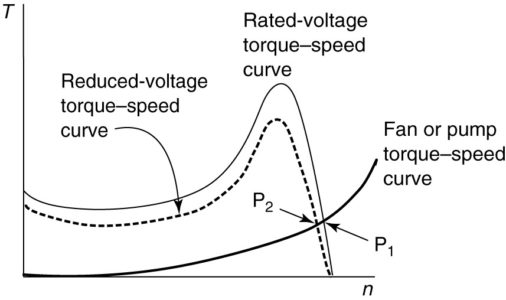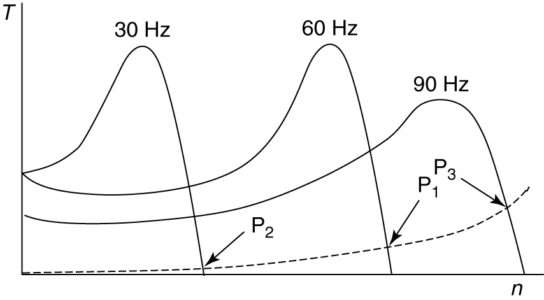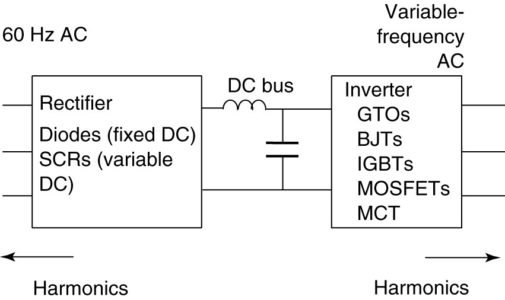Home / DC Machines / Variable-Frequency Drive Working Principle | Variable-Speed Induction Motor Drive

# Variable-Frequency Drive Working Principle | Variable-Speed Induction Motor Drive

Want create site? Find Free WordPress Themes and plugins.

The induction motor is basically a constant-speed motor, as it operates within 3% of the synchronous speed, which is determined by the frequency and number of poles in the machine:

$\begin{matrix} {{n}_{s}}=\frac{120\times f}{P} & {} & \left( 1 \right) \\\end{matrix}$

Although torque at a given slip is proportional to the voltage squared, changing the magnitude of the applied voltage has a very little effect on the operating speed of the motor.

Figure 1 shows an induction motor torque-speed curve at rated voltage. Also shown is a torque-speed curve for a load, perhaps a fan or pump, which has a speed-squared characteristic.

At rated voltage, the operating point for the motor and load will be where the motor provides exactly the torque required by the load (plus losses, of course). That point is indicated at P, in Figure 1. The power delivered to the load could be found from the horsepower-to-torque relationship:

$\begin{matrix} HP=\frac{n\times T}{5252} & {} & \left( 2 \right) \\\end{matrix}$

Where HP is the horsepower delivered, T is the torque in lb. ft, n is the RPM of the motor, and 5252 is a constant arising from unit conversions.

Reducing the voltage applied to the motor will reduce the torque provided by the motor at a given operating speed.

Figure 1 also shows a torque-speed curve for the motor at some value of reduced voltage. Once again, the operating point will be the intersection of the torque-speed curves for the motor and the load, point P2 in this case. Note that both the speed of the motor and the torque delivered by the motor have decreased. Assuming the motor was driving a fan, less air would be moved and less power would be delivered to the load. However, the change in both speed and torque from the full-voltage condition is slight.

If we wanted to reduce the output of the fan by 50%, we probably could not do it by varying the voltage applied to the motor. Thus, we clearly must do something else to significantly change the speed of the motor and its output.FIGURE 1:  Effect of varying motor voltage on operating point for a given load.

## Induction Motor Characteristics under Varying Frequency

Looking at equation 1, the synchronous speed is a function of the frequency. We also know that the induction motor will always operate at some speed below synchronous speed. Thus, if we reduce the applied frequency by 50%, the speed of the motor should also decrease by about 50%.

Figure 2 shows torque-speed curves for a motor at three frequencies, 30, 60, and 90 Hz. It is assumed that 60 Hz is the rated frequency for the motor. It turns out that when the frequency is reduced, subject to voltage constraints, we can effectively move the torque-speed curve to the left. That is, the breakdown torque and slip speed are virtually unaffected by changing the frequency.

Note that at 30 Hz, the motor runs at about half the speed it runs at 60 Hz. The operating point, P2, requires only one-fourth as much torque as was required at 60 Hz because the fan torque is proportional to the speed squared. Thus, the power output of the motor, for this load, is about one-eighth of what it would be at 60 Hz.

Thus, a variable-frequency AC motor drive allows us to change the operating condition of the load and to reduce the amount of power required when doing it. Compare that to the alternative of adding dampers to the air outlet and using full power to deliver less air when the dampers are closed down and it becomes obvious why variable-frequency drives are being widely applied.FIGURE 2:  Operating points for an induction motor at varying frequencies.

The other possibility shown in Figure 2 is operating above rated frequency.

When the frequency is increased above rated frequency, the rated torque and breakdown torque will decrease. Thus, the 90 Hz, the torque-speed curve is shown with a smaller breakdown torque. The new operating point, indicated by P3, would require substantially more power than the original point P1.

If the motor was delivering rated horsepower at 60 Hz, then it would be overloaded at 90 Hz due to the increased load. Therefore, if it is desired to operate this load above 60 Hz, the motor must be sized to handle the higher load at increased frequency.

Rectifier and Inverter Stage

Clearly, if we could provide variable-frequency power to the motor, we could handle variable loads and save energy doing it. The question is how can we change the frequency of the AC power to the motor? For many years, that was an extremely expensive proposition and variable-frequency AC motors were seldom used. Now, however, it is feasible and economical. The basic block diagram of a variable-frequency drive is shown in Figure 3.FIGURE 3:  Block diagram for a variable-frequency AC motor drive.

To create variable-frequency AC, it is first necessary to rectify the 60 Hz power from the power system to DC. The DC voltage is then inverted to create the desired frequency AC voltage. Thus, Figure 3 shows three sections of the motor drive: the rectifier, the DC bus, and the inverter.

Not shown, but vital to the operation, is a control system. The control scheme that is used determines the type of output from the inverter and the type of rectifier required.

Did you find apk for android? You can find new Free Android Games and apps.